# Chapter7 thetimevalueofmoney

18 de Nov de 2019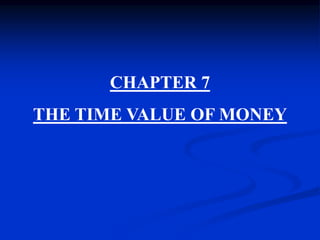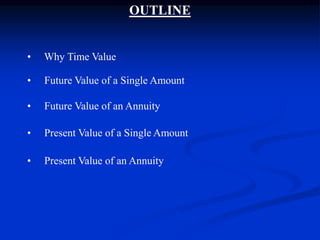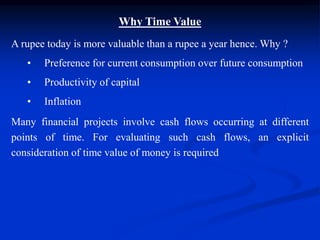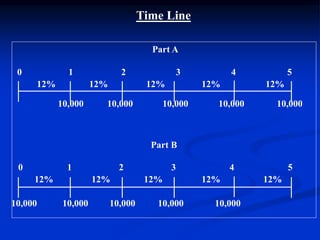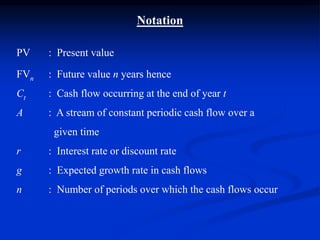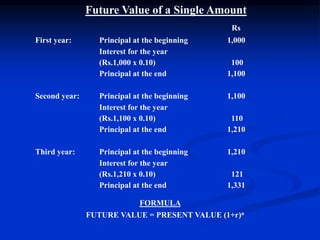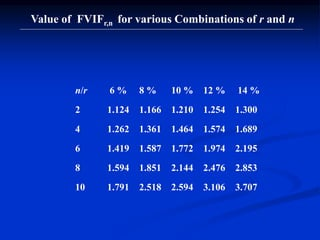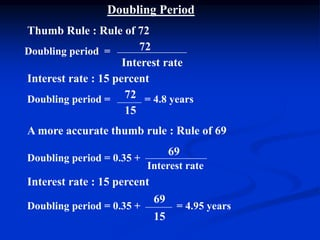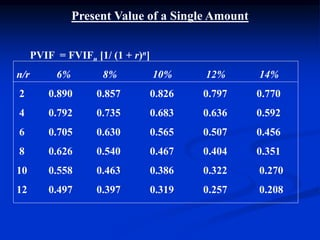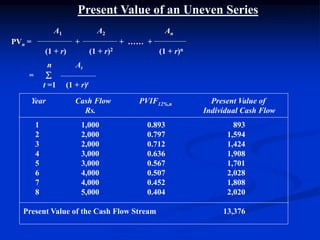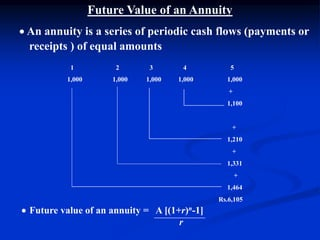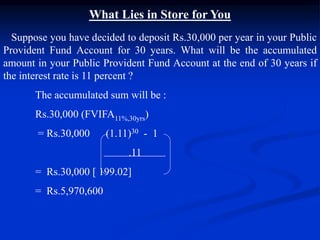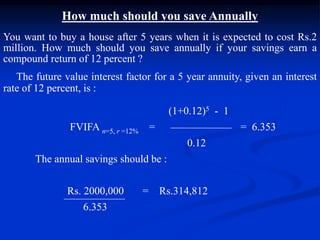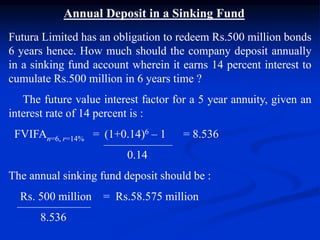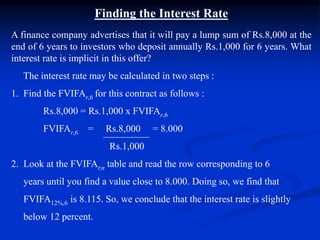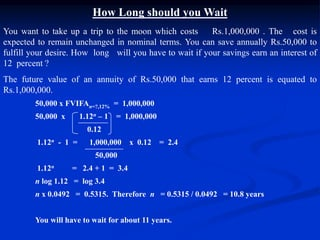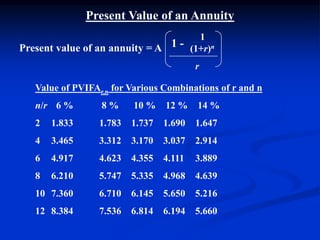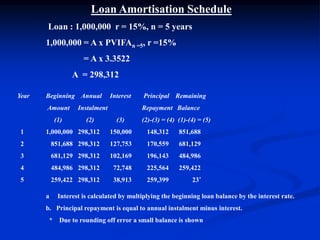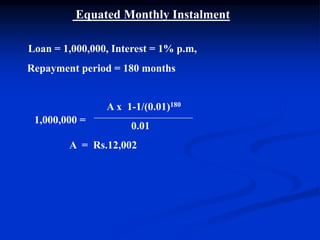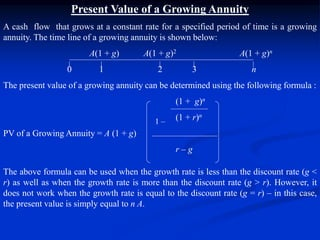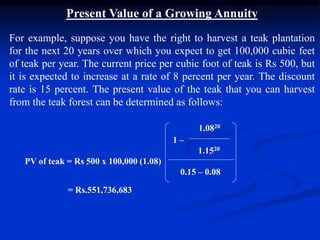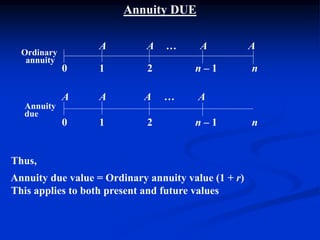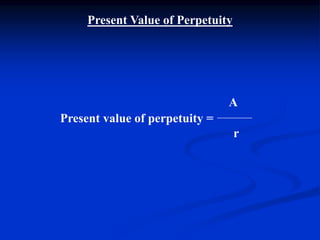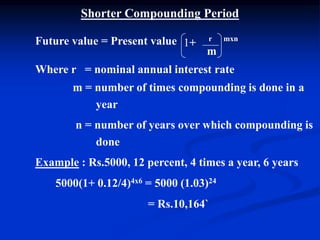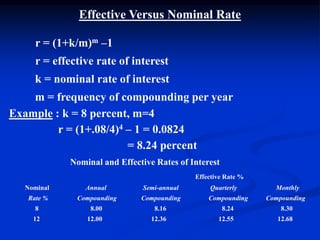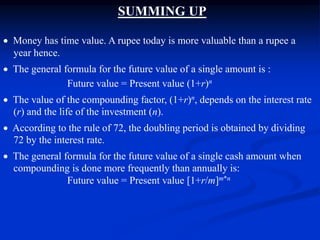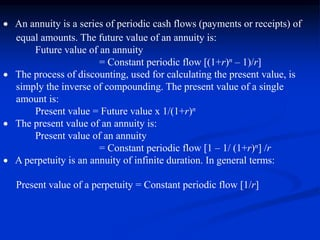1 de 27

### Chapter7 thetimevalueofmoney

• 1. CHAPTER 7 THE TIME VALUE OF MONEY  Centre for Financial Management , Bangalore
• 2. • Why Time Value • Future Value of a Single Amount • Future Value of an Annuity • Present Value of a Single Amount • Present Value of an Annuity OUTLINE
• 3. Why Time Value A rupee today is more valuable than a rupee a year hence. Why ? • Preference for current consumption over future consumption • Productivity of capital • Inflation Many financial projects involve cash flows occurring at different points of time. For evaluating such cash flows, an explicit consideration of time value of money is required
• 4. Part A 0 1 2 3 4 5 12% 12% 12% 12% 12% 10,000 10,000 10,000 10,000 10,000 Part B 0 1 2 3 4 5 12% 12% 12% 12% 12% 10,000 10,000 10,000 10,000 10,000 Time Line
• 5. PV : Present value FVn : Future value n years hence Ct : Cash flow occurring at the end of year t A : A stream of constant periodic cash flow over a given time r : Interest rate or discount rate g : Expected growth rate in cash flows n : Number of periods over which the cash flows occur Notation
• 6. Future Value of a Single Amount Rs First year: Principal at the beginning 1,000 Interest for the year (Rs.1,000 x 0.10) 100 Principal at the end 1,100 Second year: Principal at the beginning 1,100 Interest for the year (Rs.1,100 x 0.10) 110 Principal at the end 1,210 Third year: Principal at the beginning 1,210 Interest for the year (Rs.1,210 x 0.10) 121 Principal at the end 1,331 FORMULA FUTURE VALUE = PRESENT VALUE (1+r)n
• 7. n/r 6 % 8 % 10 % 12 % 14 % 2 1.124 1.166 1.210 1.254 1.300 4 1.262 1.361 1.464 1.574 1.689 6 1.419 1.587 1.772 1.974 2.195 8 1.594 1.851 2.144 2.476 2.853 10 1.791 2.518 2.594 3.106 3.707 Value of FVIFr,n for various Combinations of r and n
• 8. Doubling Period Thumb Rule : Rule of 72 72 Interest rate Interest rate : 15 percent 72 15 A more accurate thumb rule : Rule of 69 69 Interest rate Interest rate : 15 percent 69 15 Doubling period = = 4.8 yearsDoubling period = Doubling period = 0.35 + Doubling period = 0.35 + = 4.95 years
• 9. Present Value of a Single Amount PVIF = FVIFn [1/ (1 + r)n] n/r 6% 8% 10% 12% 14% 2 0.890 0.857 0.826 0.797 0.770 4 0.792 0.735 0.683 0.636 0.592 6 0.705 0.630 0.565 0.507 0.456 8 0.626 0.540 0.467 0.404 0.351 10 0.558 0.463 0.386 0.322 0.270 12 0.497 0.397 0.319 0.257 0.208
• 10. Present Value of an Uneven Series A1 A2 An PVn = + + …… + (1 + r) (1 + r)2 (1 + r)n n At =  t =1 (1 + r)t Year Cash Flow PVIF12%,n Present Value of Rs. Individual Cash Flow 1 1,000 0.893 893 2 2,000 0.797 1,594 3 2,000 0.712 1,424 4 3,000 0.636 1,908 5 3,000 0.567 1,701 6 4,000 0.507 2,028 7 4,000 0.452 1,808 8 5,000 0.404 2,020 Present Value of the Cash Flow Stream 13,376
• 11. Future Value of an Annuity  An annuity is a series of periodic cash flows (payments or receipts ) of equal amounts 1 2 3 4 5 1,000 1,000 1,000 1,000 1,000 + 1,100 + 1,210 + 1,331 + 1,464 Rs.6,105  Future value of an annuity = A [(1+r)n-1] r
• 12. What Lies in Store for You Suppose you have decided to deposit Rs.30,000 per year in your Public Provident Fund Account for 30 years. What will be the accumulated amount in your Public Provident Fund Account at the end of 30 years if the interest rate is 11 percent ? The accumulated sum will be : Rs.30,000 (FVIFA11%,30yrs) = Rs.30,000 (1.11)30 - 1 .11 = Rs.30,000 [ 199.02] = Rs.5,970,600
• 13. How much should you save Annually You want to buy a house after 5 years when it is expected to cost Rs.2 million. How much should you save annually if your savings earn a compound return of 12 percent ? The future value interest factor for a 5 year annuity, given an interest rate of 12 percent, is : (1+0.12)5 - 1 FVIFA n=5, r =12% = = 6.353 0.12 The annual savings should be : Rs. 2000,000 = Rs.314,812 6.353
• 14. Annual Deposit in a Sinking Fund Futura Limited has an obligation to redeem Rs.500 million bonds 6 years hence. How much should the company deposit annually in a sinking fund account wherein it earns 14 percent interest to cumulate Rs.500 million in 6 years time ? The future value interest factor for a 5 year annuity, given an interest rate of 14 percent is : FVIFAn=6, r=14% = (1+0.14)6 – 1 = 8.536 0.14 The annual sinking fund deposit should be : Rs. 500 million = Rs.58.575 million 8.536
• 15. Finding the Interest Rate A finance company advertises that it will pay a lump sum of Rs.8,000 at the end of 6 years to investors who deposit annually Rs.1,000 for 6 years. What interest rate is implicit in this offer? The interest rate may be calculated in two steps : 1. Find the FVIFAr,6 for this contract as follows : Rs.8,000 = Rs.1,000 x FVIFAr,6 FVIFAr,6 = Rs.8,000 = 8.000 Rs.1,000 2. Look at the FVIFAr,n table and read the row corresponding to 6 years until you find a value close to 8.000. Doing so, we find that FVIFA12%,6 is 8.115. So, we conclude that the interest rate is slightly below 12 percent.
• 16. How Long should you Wait You want to take up a trip to the moon which costs Rs.1,000,000 . The cost is expected to remain unchanged in nominal terms. You can save annually Rs.50,000 to fulfill your desire. How long will you have to wait if your savings earn an interest of 12 percent ? The future value of an annuity of Rs.50,000 that earns 12 percent is equated to Rs.1,000,000. 50,000 x FVIFAn=?,12% = 1,000,000 50,000 x 1.12n – 1 = 1,000,000 0.12 1.12n - 1 = 1,000,000 x 0.12 = 2.4 50,000 1.12n = 2.4 + 1 = 3.4 n log 1.12 = log 3.4 n x 0.0492 = 0.5315. Therefore n = 0.5315 / 0.0492 = 10.8 years You will have to wait for about 11 years.
• 17.  Centre for Financial Management , Bangalore Present Value of an Annuity 1 (1+r)n r Value of PVIFAr,n for Various Combinations of r and n n/r 6 % 8 % 10 % 12 % 14 % 2 1.833 1.783 1.737 1.690 1.647 4 3.465 3.312 3.170 3.037 2.914 6 4.917 4.623 4.355 4.111 3.889 8 6.210 5.747 5.335 4.968 4.639 10 7.360 6.710 6.145 5.650 5.216 12 8.384 7.536 6.814 6.194 5.660 1 -Present value of an annuity = A
• 18. Loan Amortisation Schedule Loan : 1,000,000 r = 15%, n = 5 years 1,000,000 = A x PVIFAn =5, r =15% = A x 3.3522 A = 298,312 Year Beginning Annual Interest Principal Remaining Amount Instalment Repayment Balance (1) (2) (3) (2)-(3) = (4) (1)-(4) = (5) 1 1,000,000 298,312 150,000 148,312 851,688 2 851,688 298,312 127,753 170,559 681,129 3 681,129 298,312 102,169 196,143 484,986 4 484,986 298,312 72,748 225,564 259,422 5 259,422 298,312 38,913 259,399 23* a Interest is calculated by multiplying the beginning loan balance by the interest rate. b. Principal repayment is equal to annual instalment minus interest. * Due to rounding off error a small balance is shown
• 19. Equated Monthly Instalment Loan = 1,000,000, Interest = 1% p.m, Repayment period = 180 months A x 1-1/(0.01)180 0.01 A = Rs.12,002 1,000,000 =
• 20. Present Value of a Growing Annuity A cash flow that grows at a constant rate for a specified period of time is a growing annuity. The time line of a growing annuity is shown below: A(1 + g) A(1 + g)2 A(1 + g)n 0 1 2 3 n The present value of a growing annuity can be determined using the following formula : (1 + g)n (1 + r)n PV of a Growing Annuity = A (1 + g) r – g The above formula can be used when the growth rate is less than the discount rate (g < r) as well as when the growth rate is more than the discount rate (g > r). However, it does not work when the growth rate is equal to the discount rate (g = r) – in this case, the present value is simply equal to n A. 1 –
• 21. Present Value of a Growing Annuity For example, suppose you have the right to harvest a teak plantation for the next 20 years over which you expect to get 100,000 cubic feet of teak per year. The current price per cubic foot of teak is Rs 500, but it is expected to increase at a rate of 8 percent per year. The discount rate is 15 percent. The present value of the teak that you can harvest from the teak forest can be determined as follows: 1.0820 1 – 1.1520 PV of teak = Rs 500 x 100,000 (1.08) 0.15 – 0.08 = Rs.551,736,683
• 22. Annuity DUE A A … A A 0 1 2 n – 1 n A A A … A 0 1 2 n – 1 n Thus, Annuity due value = Ordinary annuity value (1 + r) This applies to both present and future values Ordinary annuity Annuity due
• 23. Present Value of Perpetuity A Present value of perpetuity = r
• 24. Shorter Compounding Period Future value = Present value r mxn m Where r = nominal annual interest rate m = number of times compounding is done in a year n = number of years over which compounding is done Example : Rs.5000, 12 percent, 4 times a year, 6 years 5000(1+ 0.12/4)4x6 = 5000 (1.03)24 = Rs.10,164` 1+
• 25. Effective Versus Nominal Rate r = (1+k/m)m –1 r = effective rate of interest k = nominal rate of interest m = frequency of compounding per year Example : k = 8 percent, m=4 r = (1+.08/4)4 – 1 = 0.0824 = 8.24 percent Nominal and Effective Rates of Interest Effective Rate % Nominal Annual Semi-annual Quarterly Monthly Rate % Compounding Compounding Compounding Compounding 8 8.00 8.16 8.24 8.30 12 12.00 12.36 12.55 12.68
• 26. SUMMING UP  Money has time value. A rupee today is more valuable than a rupee a year hence.  The general formula for the future value of a single amount is : Future value = Present value (1+r)n  The value of the compounding factor, (1+r)n, depends on the interest rate (r) and the life of the investment (n).  According to the rule of 72, the doubling period is obtained by dividing 72 by the interest rate.  The general formula for the future value of a single cash amount when compounding is done more frequently than annually is: Future value = Present value [1+r/m]m*n
• 27.  An annuity is a series of periodic cash flows (payments or receipts) of equal amounts. The future value of an annuity is: Future value of an annuity = Constant periodic flow [(1+r)n – 1)/r]  The process of discounting, used for calculating the present value, is simply the inverse of compounding. The present value of a single amount is: Present value = Future value x 1/(1+r)n  The present value of an annuity is: Present value of an annuity = Constant periodic flow [1 – 1/ (1+r)n] /r  A perpetuity is an annuity of infinite duration. In general terms: Present value of a perpetuity = Constant periodic flow [1/r]  Centre for Financial Management , Bangalore